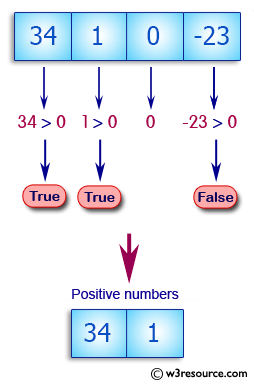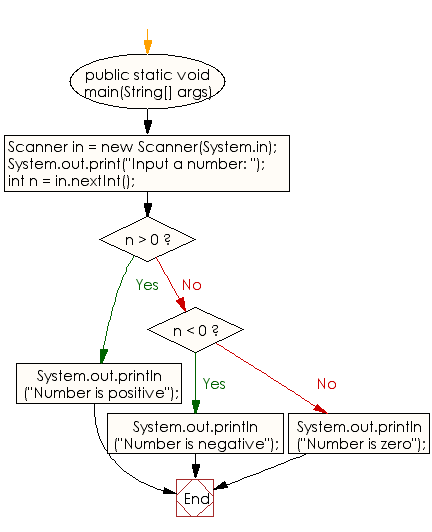﻿ Java exercises: Check the number is negative, zero, or positive - w3resource# Java Conditional Statement Exercises: Check the number is negative, zero, or positive

## Java Conditional Statement: Exercise-27 with Solution

Write a Java program that reads an integer and check whether it is negative, zero, or positive.

Test Data
Input a number: 7

Pictorial Presentation:Sample Solution:

Java Code:

``````import java.util.Scanner;
public class Exercise27 {
public static void main(String[] args)
{
Scanner in = new Scanner(System.in);
System.out.print("Input a number: ");
int n = in.nextInt();

if (n > 0)
{
System.out.println("Number is positive");
}
else if (n < 0)
{
System.out.println("Number is negative");
}
else
{
System.out.println("Number is zero");
}
}
}
```
```

Sample Output:

```Input a number: 7
Number is positive
```

Flowchart:Java Code Editor:

What is the difficulty level of this exercise?

﻿

## Java: Tips of the Day

How to split a String by space?

You can use the whitespace regex:

```str = "Hello I'm your String";
String[] splited = str.split("\\s+");
```

This will cause any number of consecutive spaces to split your string into tokens.

Ref: https://bit.ly/3xk42If